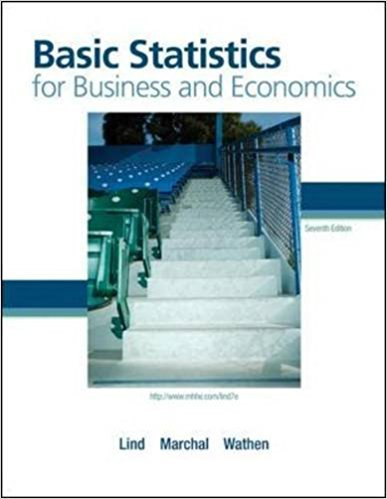×
×

# Consider the following chart. a. What is this chartISBN: 9780077384470 64

## Solution for problem 1E Chapter 4

Basic Statistics for Business and Economics | 7th Edition

• Textbook Solutions
• 2901 Step-by-step solutions solved by professors and subject experts
• Get 24/7 help from StudySoup virtual teaching assistantsBasic Statistics for Business and Economics | 7th Edition

4 5 1 344 Reviews
30
2
Problem 1E

Consider the following chart.a. What is this chart called?

b. How many observations are in the study?

c. What are the maximum and the minimum values?

d. Around what values do the observations tend to cluster?

Step-by-Step Solution:

Step 1 of 4:

Given a chart.Our goal is :

a). We need to find the chart.

b). We need to find observations.

c). We need to find the maximum and the minimum values.

d). We need to find the what values do the observations tend to cluster.

a). This chart is called dot plot.

Step 2 of 4:

b). There are 7 observations are in the study.

Step 3 of 4

Step 4 of 4

##### ISBN: 9780077384470

This full solution covers the following key subjects: values, Observations, chart, maximum, consider. This expansive textbook survival guide covers 6 chapters, and 325 solutions. Basic Statistics for Business and Economics was written by and is associated to the ISBN: 9780077384470. Since the solution to 1E from 4 chapter was answered, more than 289 students have viewed the full step-by-step answer. The full step-by-step solution to problem: 1E from chapter: 4 was answered by , our top Business solution expert on 08/23/17, 08:36AM. The answer to “Consider the following chart. a. What is this chart called?________________b. How many observations are in the study?________________c. What are the maximum and the minimum values?________________d. Around what values do the observations tend to cluster?” is broken down into a number of easy to follow steps, and 34 words. This textbook survival guide was created for the textbook: Basic Statistics for Business and Economics , edition: 7.

Unlock Textbook Solution